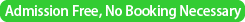### Seminar on "Stochastic Models and Discrete Geometry"

Date
October 7, 2011Place
Room D312A, The Institute of Statistical Mathematics,
Tachikawa, Tokyo
Program

10:30-11:10
Psi-series method for random trees and quadratic convolution recurrences

By Hsien-Kuei Hwang
(Institute of Statistical Science, Academia Sinica, Taipei)

11:30-12:10
Enumeration of 3D triangular maps for creation of 3D universe

By Tetsuyuki Yukawa (Sokendai and KEK)

12:30-1:30
Lunch

1:30-2:10
Duality between population and sample in interacting particle systems on graphs

By Shuhei Mano (ISM and Sokendai)

2:30- 3:00
Free talking

3:00-3:30
Tea

(Supported by ISM Cooperative ResearchProgram 2011- ISM・CRP 1023 and JSPS Grant-in-aid for Scientific Research 23540177).

Speaker 1
Hsien-Kuei Hwang
(Institute of Statistical Science, Academia Sinica, Taipei)
Title
Psi-series method for random trees and quadratic convolution recurrences
Abstract

An unusual and surprising expansion of the form $p_n = \rho^{-n-1}\left(6n +\tfrac{18}5+ \tfrac{336}{3125} n^{-5}+\tfrac{1008}{3125}\, n^{-6} +\text{smaller order terms}\right),$

as $n\to\infty$, is derived for the probability $p_n$ that two randomly chosen binary search trees are identical (in shape, hence in labels of all corresponding nodes). A quantity arising in the analysis of phylogenetic trees is also proved to have a similar asymptotic expansion. Our method of proof is new in the literature of discrete probability and the analysis of algorithms, and it is based on the logarithmic psi-series expansions for nonlinear differential equations. Such an approach is very general and applicable to many other problems involving nonlinear differential equations; many examples are discussed in this article and several attractive phenomena are discovered.

Speaker 2
Tetsuyuki Yukawa (Sokendai and KEK)
Title
Enumeration of 3D triangular maps for creation of 3D universe
Abstract
Enumeration of planar maps has been extensively studied both in mathematics and physics, and recently in computer science. I have been working in cosmology, where enumeration of space time geometry is my main task. I have constructed the master equation for the birth and growth of universe, in which the enumeration of triangular maps plays an important role as the potential of motive force for the expansion of universe. Recently, I made a detailed analysis for the 2D universe by making use of the Tutte theory together with the conformal field theory.
My ultimate goal is the evolution of our universe which is at least 4- dimensional. For the next step I started to study 3D triangulation maps by extending the Tutte theory. I would like to ask your help and opinion on the topics through exhibiting my struggle on this subject.
Speaker 3
Shuhei Mano (ISM and Sokendai)
Title
Duality between population and sample in interacting particle systems on graphs
Abstract
It is well known that duality of Markov chain is very useful to analyze interacting particle systems. In this talk, two interacting particle systems, whose diffusive limit have a dual process which generates a random graph which represents the structure of the sample, will be presented. I would like to demonstrate that the duality is useful to analyse structured sample from a population which obeys complex probability model. One of the models is a graph-valued interacting particle system introduced by Itoh, Mallows, Shepp (1998). The Markov process of number of particles which take a value of a node of the graph. Two particles are randomly chosen, and if these particles have neighboring values one of them change its value to the other particle. For a complete graph the dual is Kingman's coalescent. But in general the dual is a random collision model.
Properties of samples taken from the population are discussed, including a Martingale which was effectively used to obtain absorption probability of the diffusion by Itoh et al. The other model is a biased voter model, in which a set of complete graphs and each graph have many sites which takes states of 0 or 1. The state of each particle randomly follows states of other sites. Properties of samples are discussed, and an importance sampling with a perfect simulation is presented to solve a system of linear recursion of the likelihood. The model is also an extension of the Kingman's coalescent and could be regarded as a model of biased gene conversion is genomes.